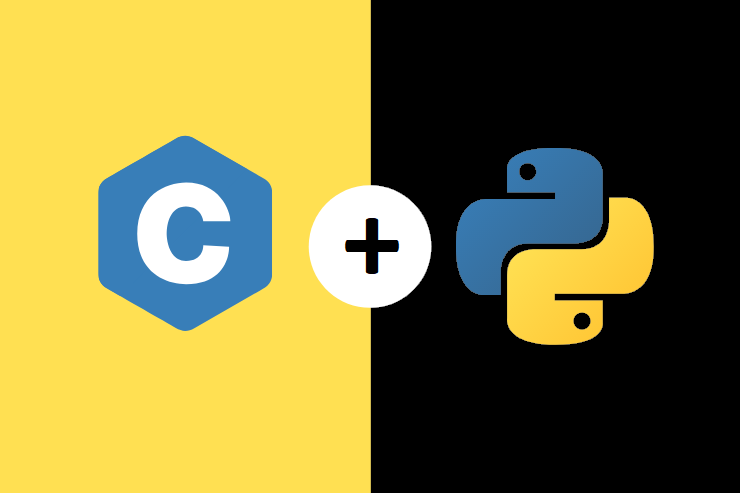# Import C Code in Python (Part 1)## Importing C libraries and functions in Python using ctypes library and Cython

C is known by its speed, so sometimes when the performance and the speed are an issue, developers go to write complex functions or loops in C to get the benefits of a low-level language.

We will explore two ways to run C code from within Python. We will achieve that using two methods: ctypes library and Cython language which is a superset of python that compiles into C.

In part 1 of the post we will explore ctypes. While in part 2, we will do the task with Cython.

### Read C Shared Libraries into Python with Ctypes

We will begin first with the ctypes library. Ctypes enables reading C shared libraries in python and using the predefined functions just any python functions.

The advantage of using ctypes is that it is simple. But on the other hand, it has some limitation especially when it comes to dynamic memory allocation e.g. malloc in C.

Let’s get started!

We have written a C function that perfoms range binary search and stored it into “binary_search.c” file

```#include <stdio.h>
#include <stdlib.h>

void swap(int * x, int * y) {
int tmp = * x;
* x = * y;
* y = tmp;
}

void sorted(int * array, size_t len) {
int i, j, min_idx;

for (i = 0; i < len - 1; i++) {
min_idx = i;
for (j = i + 1; j < len; j++)
if (array[j] < array[min_idx])
min_idx = j;

swap( & array[min_idx], & array[i]);
}
}

void range_binary_search(int * array, int len, int val, int * res) {
int right = 0;
int left = 0;
int middle;

if (val < array || val > array[len - 1]) {
right = 0;
left = -1;
}

// found
else {
while (right < len) {
middle = (right + len) / 2;
if (val < array[middle])
len = middle;
else
right = middle + 1;
}
len = right - 1;
while (left < len) {
middle = (left + len) / 2;
if (val > array[middle])
left = middle + 1;
else
len = middle;
}
}
res = left;
res = right - 1;
}```

Then we have made our file a shared library

`gcc -fPIC -shared -o libbsearch.so binary_search.c`

N.B. The .so extension because I am on linux. In case of Windows it should be a .dll object.

Now, we would like to read this library into our python script and use normally the range_binary_search function.

In python, we will begin by defining a wrapper function to convert C functions from the library into python functions:

```import ctypes

def wrap_function(lib, funcname, restype, argtypes):
"""Simplify wrapping ctypes functions
func = lib.__getattr__(funcname)
func.argtypes = argtypes
func.restype = restype
return func```

Then we read the shared library

```lib = ctypes.CDLL("./libbsearch.so")
```

Now, before starting anything, we define a function to convert a python list into a C array (Pointer to Int)

```## To convert a full array
def to_cpointer(pyarr):
n = len(pyarr)
c_type = ctypes.c_int * n
c_arr = c_type(*pyarr)
return c_arr

## To initiate an empty C array
def empty_arr_init(size):
# cast the empty arr into a c_int
return (ctypes.c_int * size)()```

Now we start importing the functions from the library using the wrapper function

```c_sort = wrap_function(lib, "sorted", None,
(ctypes.POINTER(ctypes.c_int), ctypes.c_int))

bsearch = wrap_function(lib, "range_binary_search", None,
[ctypes.POINTER(ctypes.c_int), ctypes.c_int,
ctypes.c_int, ctypes.POINTER(ctypes.c_int)])```

Look how we use ctypes.POINTER because the functions use values passed by reference.

Finally a wrapper function to print the results

```def binary_search(arr, size, val, res):
bsearch(arr, size, val, res)
print("%s is at index %s till index %s" % (val, res, res))```

Now let’s test everything:

```# random unsorted list
arr = [4, 5, 5, 3, 2, 3, 7, 9, 10, 0, 11, 17, 25]
n = ctypes.c_int(len(arr))

# convert python list to c array
carr = to_cpointer(arr)
# initialize an empty c array (2 because function returns range(left, right))
res = empty_arr_init(2)

# Sort the array
c_sort(carr, n)```

Examples:

```binary_search(carr, n, 2, res)
# 2 is at index 1 till index 1

binary_search(carr, n, 3, res)
# 3 is at index 2 till index 3

binary_search(carr, n, 17, res)
# 17 is at index 11 till index 11